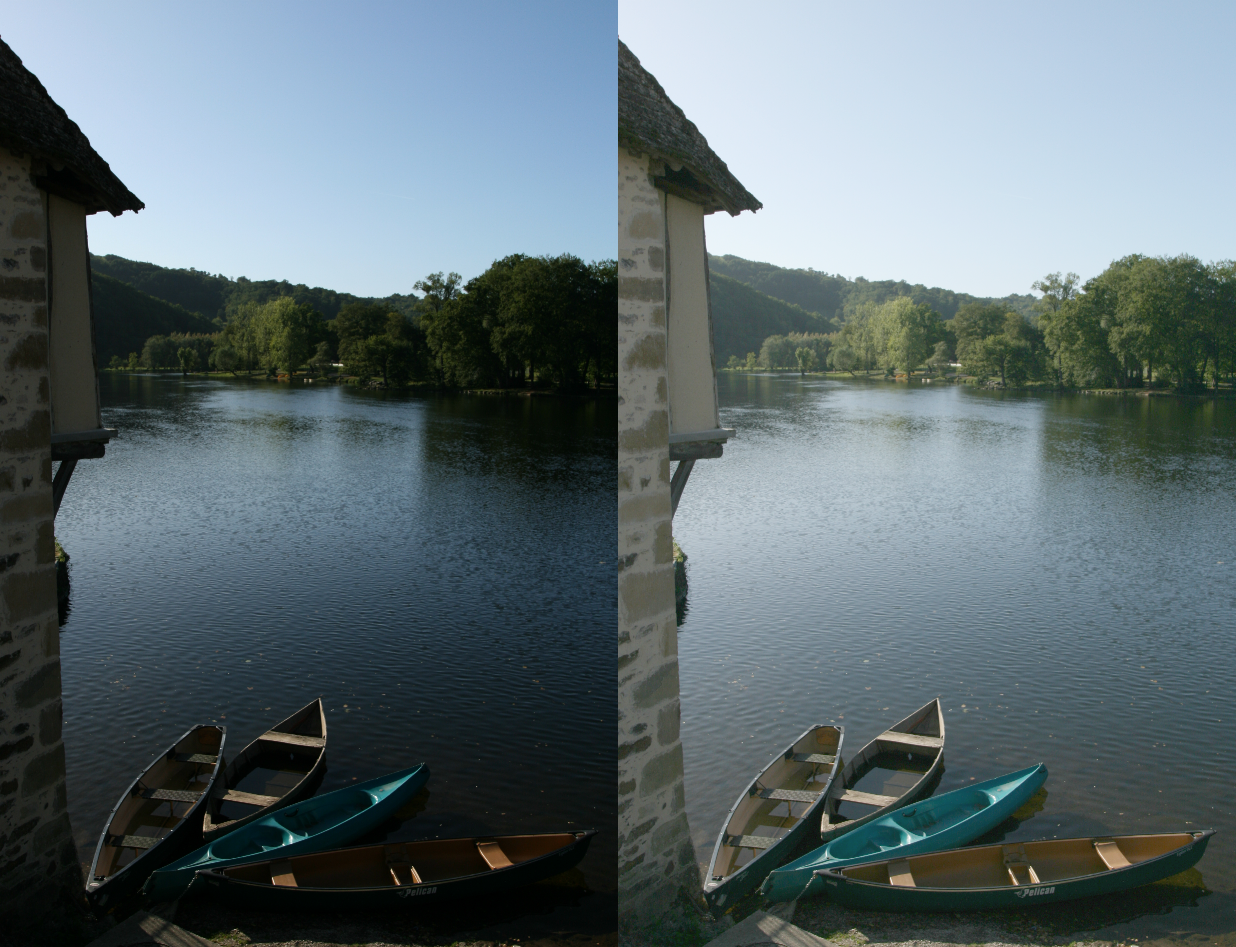Defined in 1985 by Michel Jourlin, the LIP Model (Logarithmic Image Processing) is a mathematical and physical framework dedicated to image processing. First defined as a framework adapted to images acquired in transmitted light, the interest of using LIP operators in a reflected light model has then been established.
The LIP model is based on the transmittance law. The addition law of the LIP model can be represented as the « addition » of two obstacles placed between a source and a sensor. From this addition law, LIP subtraction and LIP multiplication have been defined.
Since, many applications have been developped around these basic operators : LIP contrasts, LIP interpolation, LIP metrics, …
This plugin proposes the three basic operators : the LIP addition/subtraction of a constant to an image and the LIP scalar product of an image by a scalar. Depending on the operator, the resulting images consist of brightened or darkened versions of the original image by simulating source or sensor variations (addition/subtraction) or applying a non linear transform (multiplication).